# How to Calculate and Solve for Marhoun’s Gas Solubility in a Fluid | The Calculator Encyclopedia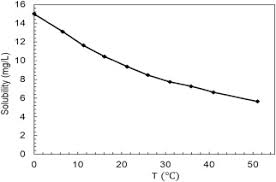The image above represents marhoun’s gas solubility.

To compute for the marhoun’s gas solubility, four essential parameters are needed and these parameters are Gas Specific Gravity (γg), Temperature (°Rankine) (T), Pressure (P) and Stock Tank Oil Gravity (γo).

The formula for calculating the marhoun’s gas solubility:

Rs = [a γgb γoc Td P]e

Where:

Rs = Marhoun’s Gas Solubility
a = 185.843208
b = 1.877840
c = -3.1437
d = -1.32657
e = 1.398441
γg = Gas Specific Gravity
T = Temperature (°Rankine)
P = Pressure
γo = Stock Tank Oil Gravity

Let’s solve an example;
Find the marhoun’s gas solubility when the gas specific gravity is 14, temperature is 80, pressure is 40 and the stock tank oil gravity is 24.

This implies that;

γg = Gas Specific Gravity = 14
T = Temperature (°Rankine) = 80
P = Pressure = 40
γo = Stock Tank Oil Gravity = 24

Rs = [a γgb γoc Td P]e
Rs = [185.843208 x 141.87784 x 24-3.1437 x 80-1.32657 x 40]1.398441
Rs = [185.843208 x 141.98 x 24-3.1437 x 80-1.32657 x 40]1.398441
Rs = [185.843208 x 141.98 x 0.0000458 x 80-1.32657 x 40]1.398441
Rs = [185.843208 x 141.98 x 0.0000458 x 0.00298 x 40]1.398441
Rs = [0.1445]1.398441
Rs = 0.0668

Therefore, the marhoun’s gas solubility is 0.0668.

Nickzom Calculator – The Calculator Encyclopedia is capable of calculating the marhoun’s gas solubility.

To get the answer and workings of the marhoun’s gas solubility using the Nickzom Calculator – The Calculator Encyclopedia. First, you need to obtain the app.

You can get this app via any of these means:

To get access to the professional version via web, you need to register and subscribe for NGN 1,500 per annum to have utter access to all functionalities.
You can also try the demo version via https://www.nickzom.org/calculator

Android (Paid) – https://play.google.com/store/apps/details?id=org.nickzom.nickzomcalculator
Android (Free) – https://play.google.com/store/apps/details?id=com.nickzom.nickzomcalculator
Apple (Paid) – https://itunes.apple.com/us/app/nickzom-calculator/id1331162702?mt=8
Once, you have obtained the calculator encyclopedia app, proceed to the Calculator Map, then click on Petroleum under EngineeringNow, Click on Fluid Properties under Petroleum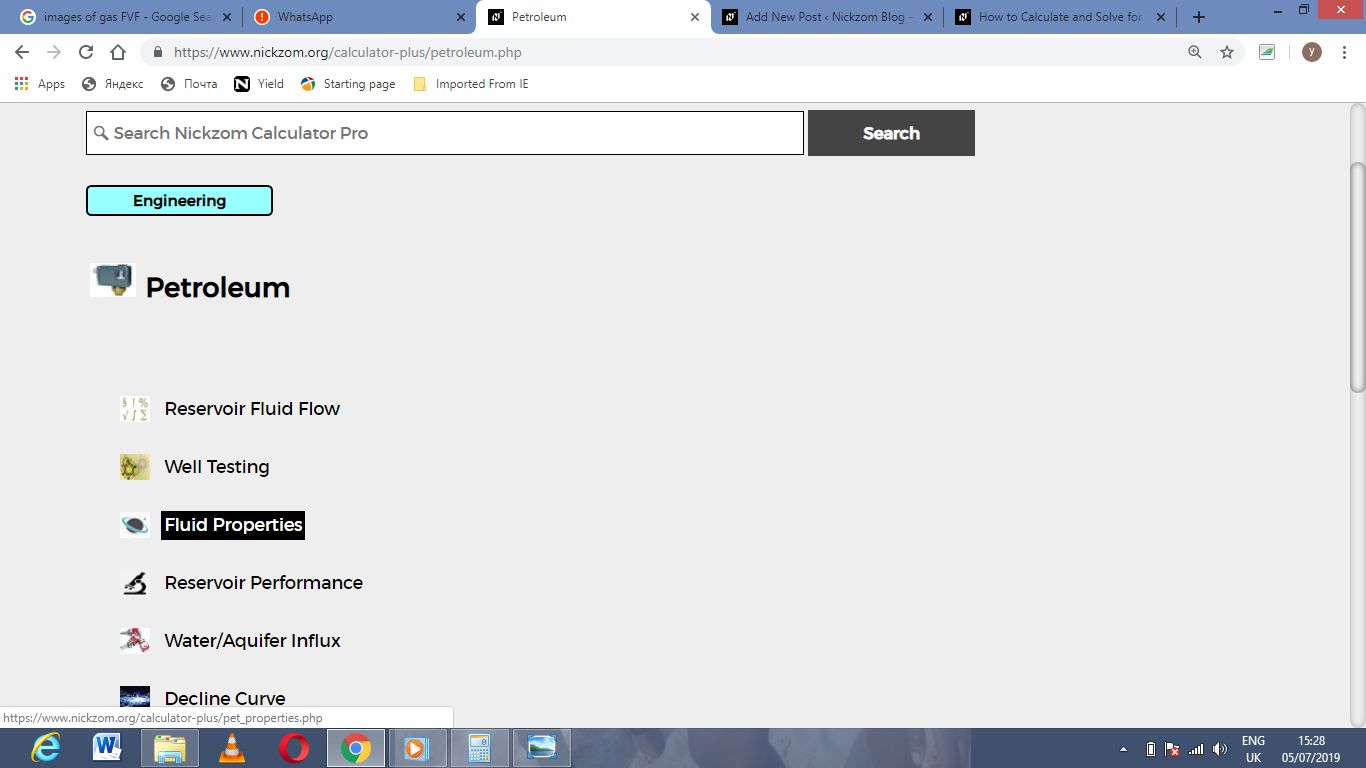Now, Click on Marhoun’s Gas Solubility under Fluid Properties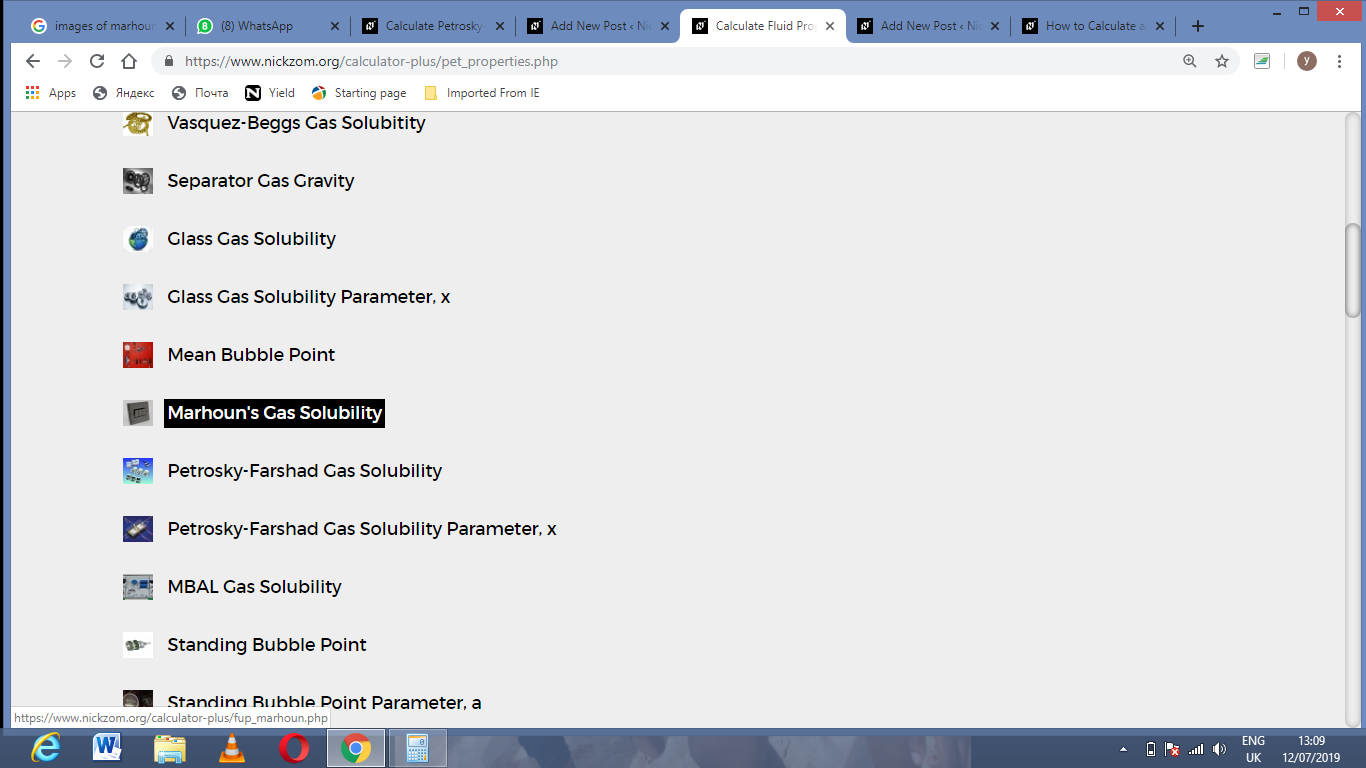The screenshot below displays the page or activity to enter your values, to get the answer for the marhoun’s gas solubility according to the respective parameters which are the Gas Specific Gravity (γg), Temperature (°Rankine) (T), Pressure (P) and Stock Tank Oil Gravity (γo).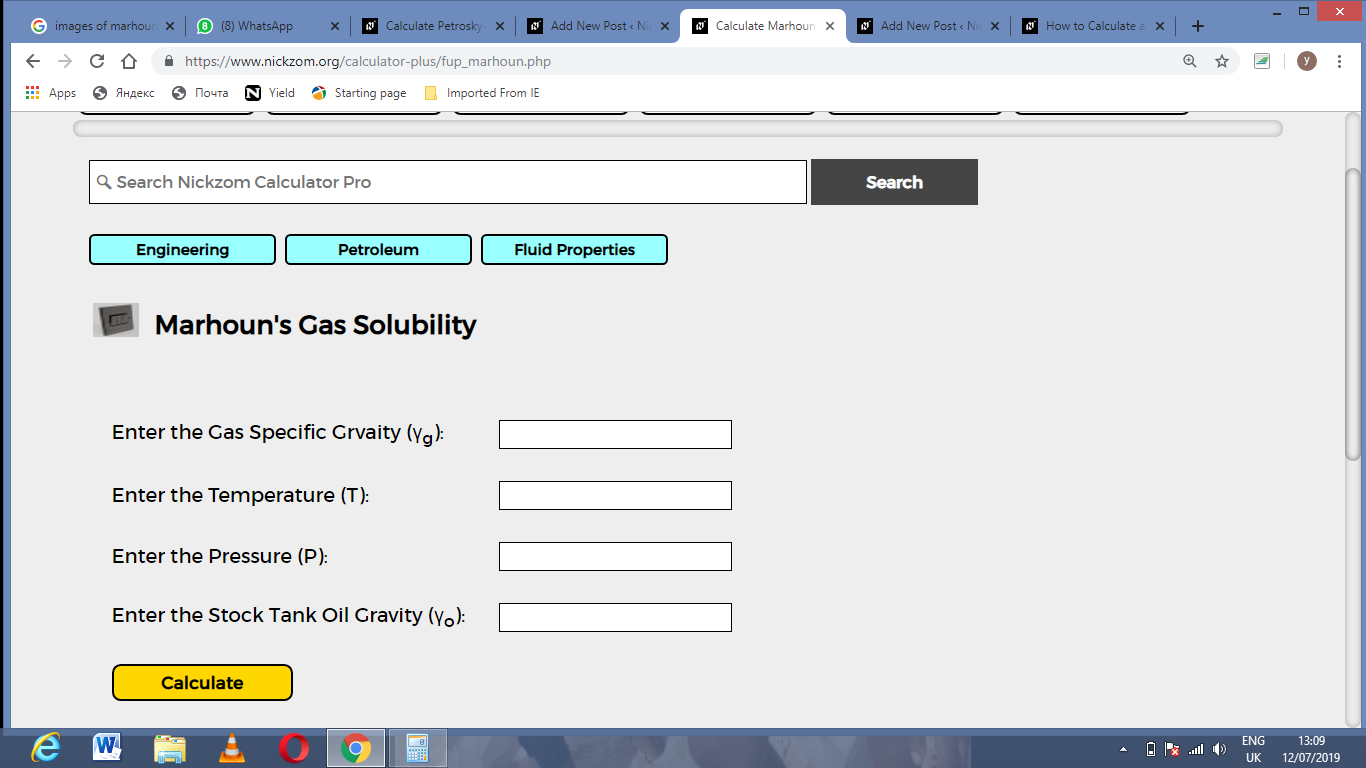Now, enter the values appropriately and accordingly for the parameters as required by the Gas Specific Gravity (γg) is 14, Temperature (°Rankine) (T) is 80, Pressure (P) is 40 and Stock Tank Oil Gravity (γo) is 24.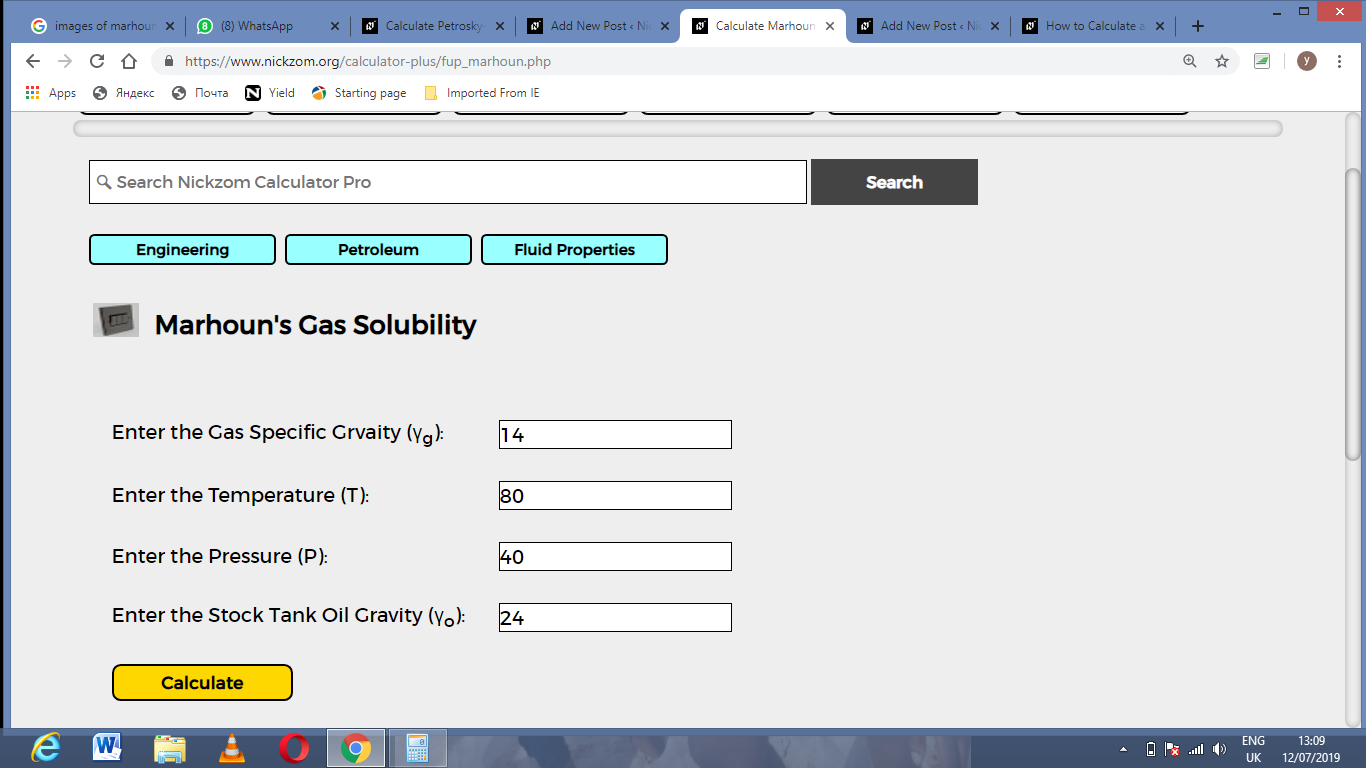Finally, Click on Calculate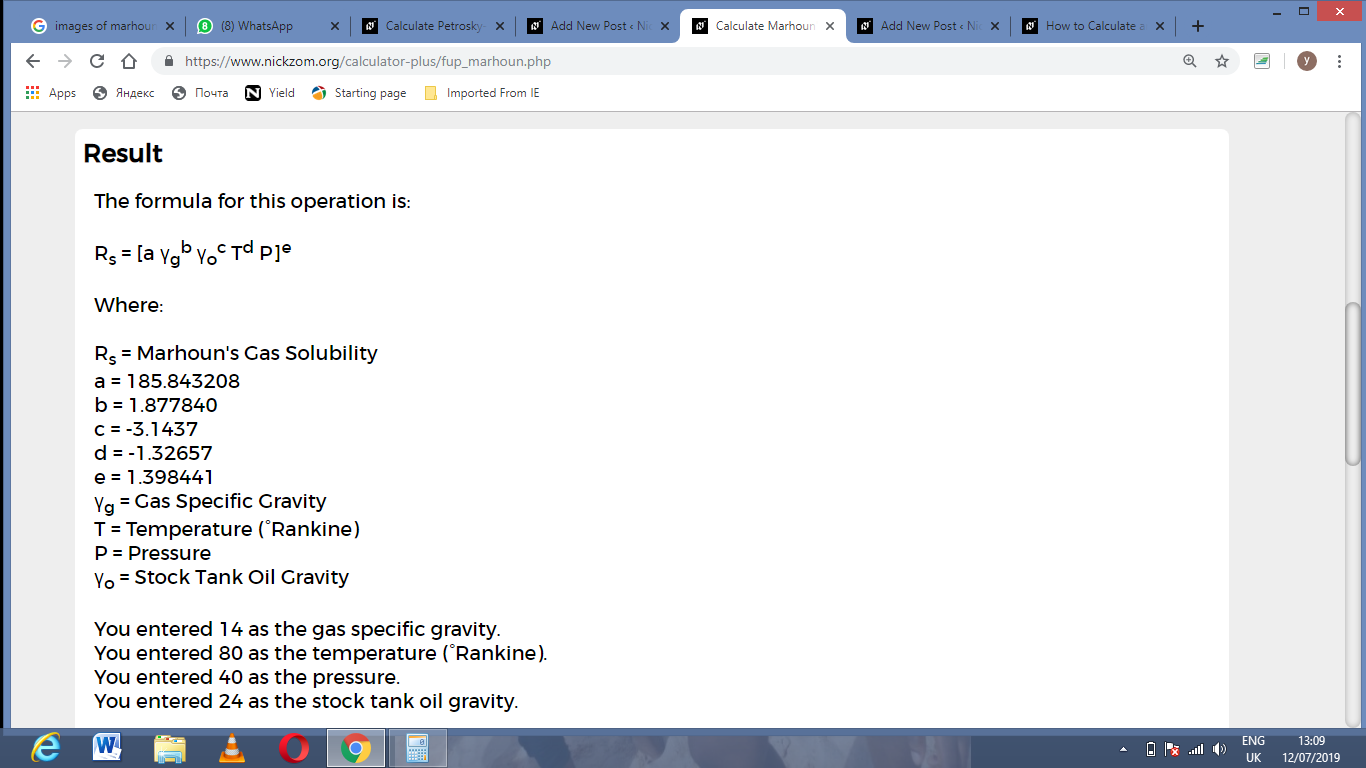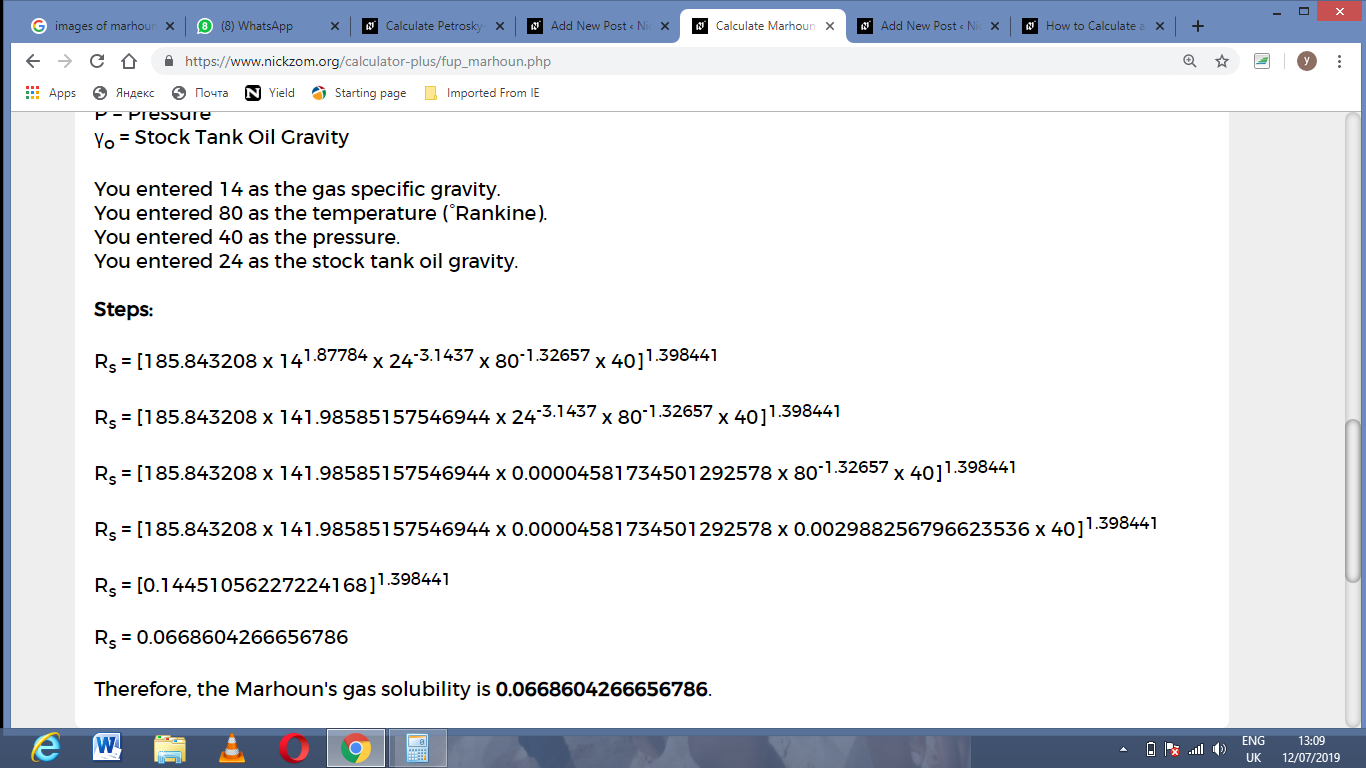As you can see from the screenshot above, Nickzom Calculator– The Calculator Encyclopedia solves for the marhoun’s gas solubility and presents the formula, workings and steps too.